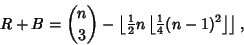## Schwenk's Formula

Letbe the number of Monochromatic Forced Triangles (whereandare the number of red and blue Triangles) in an Extremal Graph. Thenwhereis a Binomial Coefficient andis the Floor Function (Schwenk 1972).

Schwenk, A. J. Acquaintance Party Problem.'' Amer. Math. Monthly 79, 1113-1117, 1972.